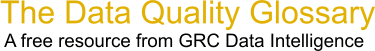Choose Index below for a list of all words and phrases defined in this glossary.

# Confidence Interval / Confidence Interval of the Mean

index | Index

## Confidence interval / Confidence Interval of the Mean - definitions

Confidence interval, or confidence interval of the mean - The upper and lower limits or values, or bounds on either side of a sample mean for which a confidence level is valid.

[Category=Data Quality ]

Source: Larry English, http://www.iaidq.com/main/glossary.shtml, 16-Jan-2009 10:52

These advertisers support this free service

Confidence Band (or Interval) - Measurement of the certainty of the shape of the fitted regression line. A 95% confidence band implies a 95% chance that the true regression line fits within the confidence bands. Measurement of certainty.

[Category=Data Quality ]

Confidence Interval - How "wide" you have to cast your "net" to be sure of capturing the true population parameter. If my estimate of defects is 10%, I might also say that my 95% Confidence Interval is plus or minus 2%, meaning that odds are 95 out of 100 hundred that the true population parameter is somewhere between 8 and 12%.

Source: iSixSigma, 08 January 2011 08:05:58, https:web.archive.org/web/20111109014246/http:www.isixsigma.com/index.php?option=com_glossaryConfidence Interval - The numerical interval constructed around a point estimate of a population parameter, combined with a probability statement (the confidence coefficient) linking it to the population's true parameter value. If the same confidence interval construction technique and assumptions are used to calculate future intervals, they will include the unknown population parameter with the same specified probability.

[Category=Quality Assurance ]

Source: Massachusetts Department of Environmental Protection, 17 March 2011 07:25:38, http://www.mass.gov/dep/cleanup/laws/glossa01.htmData Quality Glossary.  A free resource from GRC Data Intelligence. For comments, questions or feedback: dqglossary@grcdi.nl